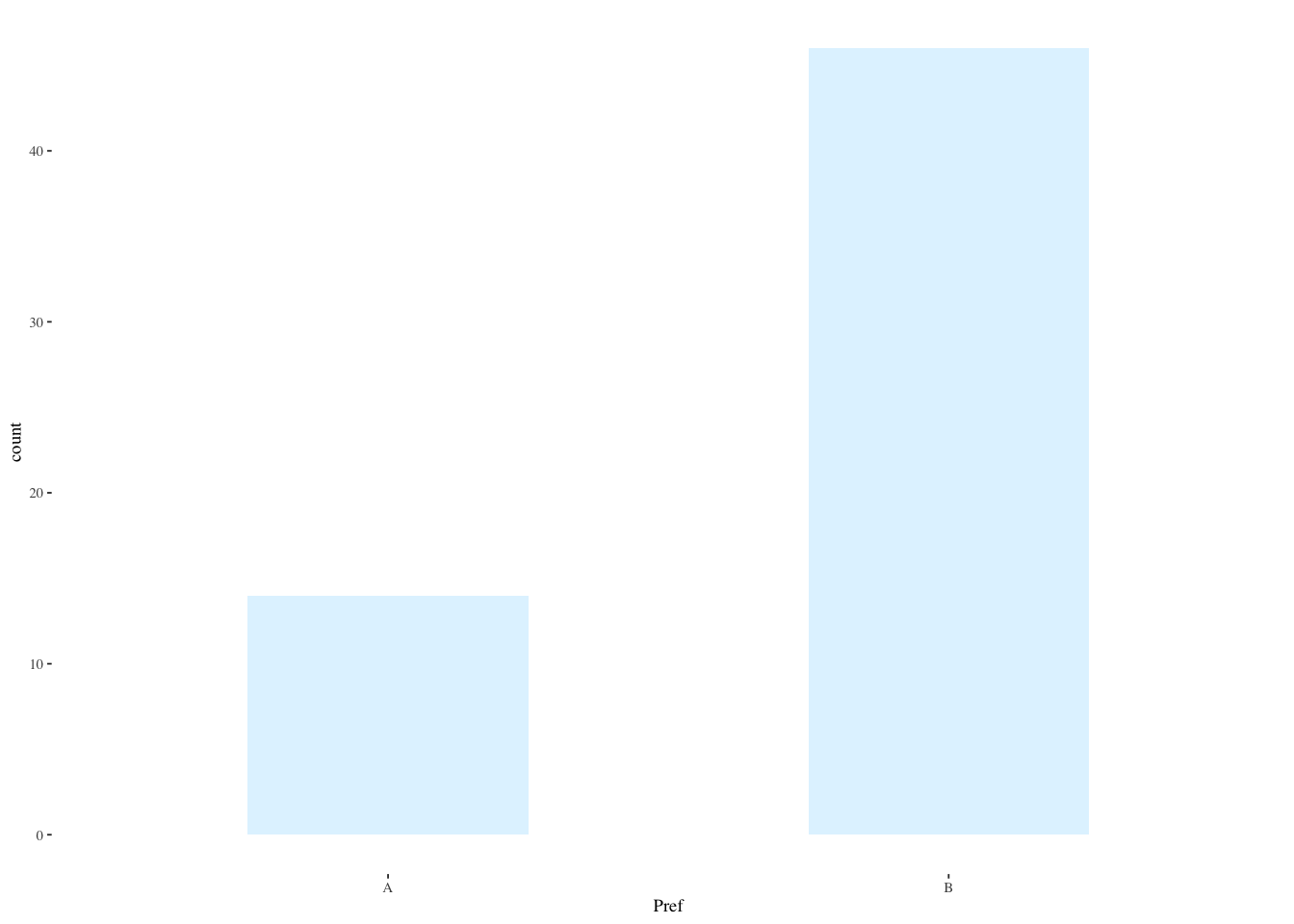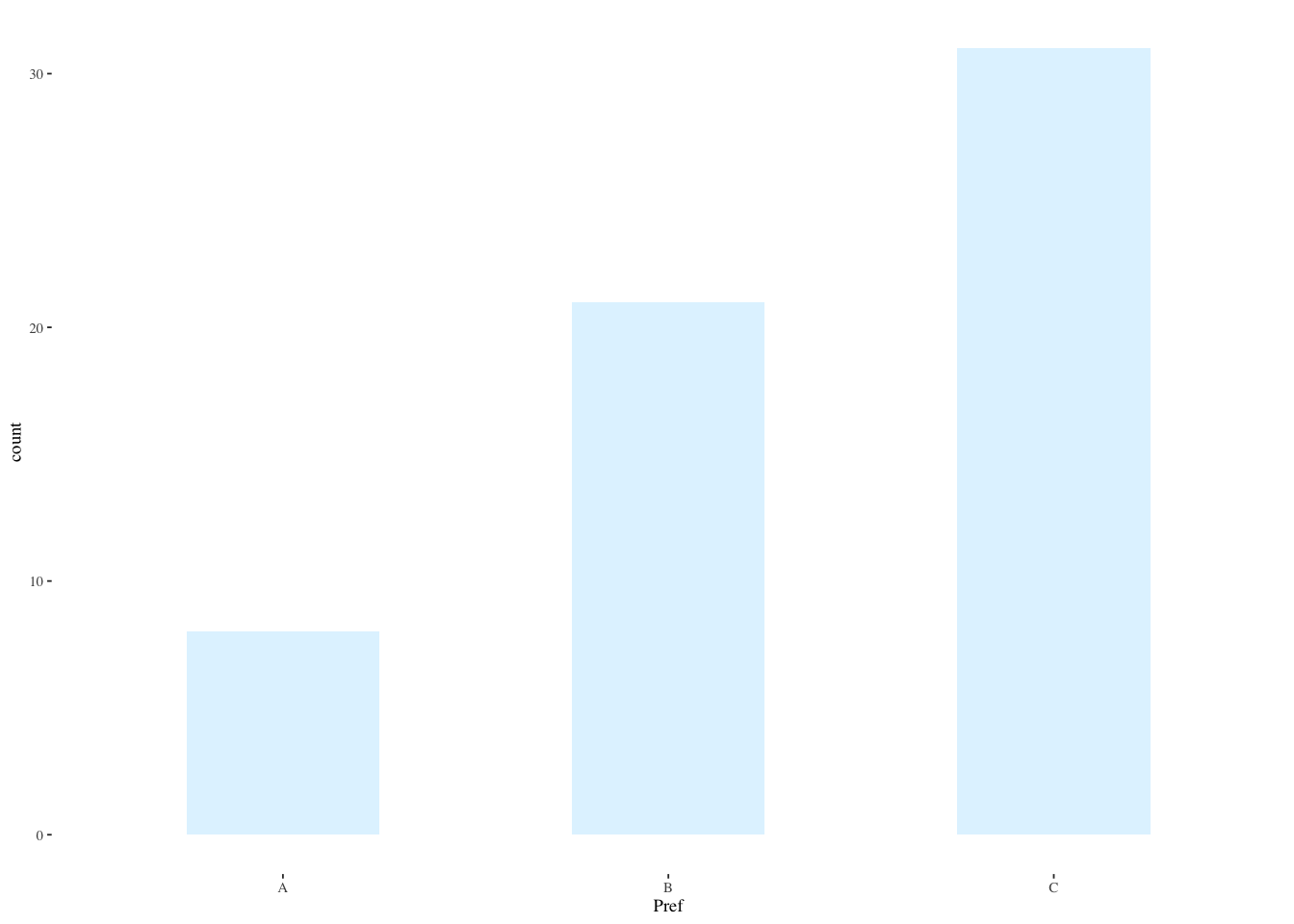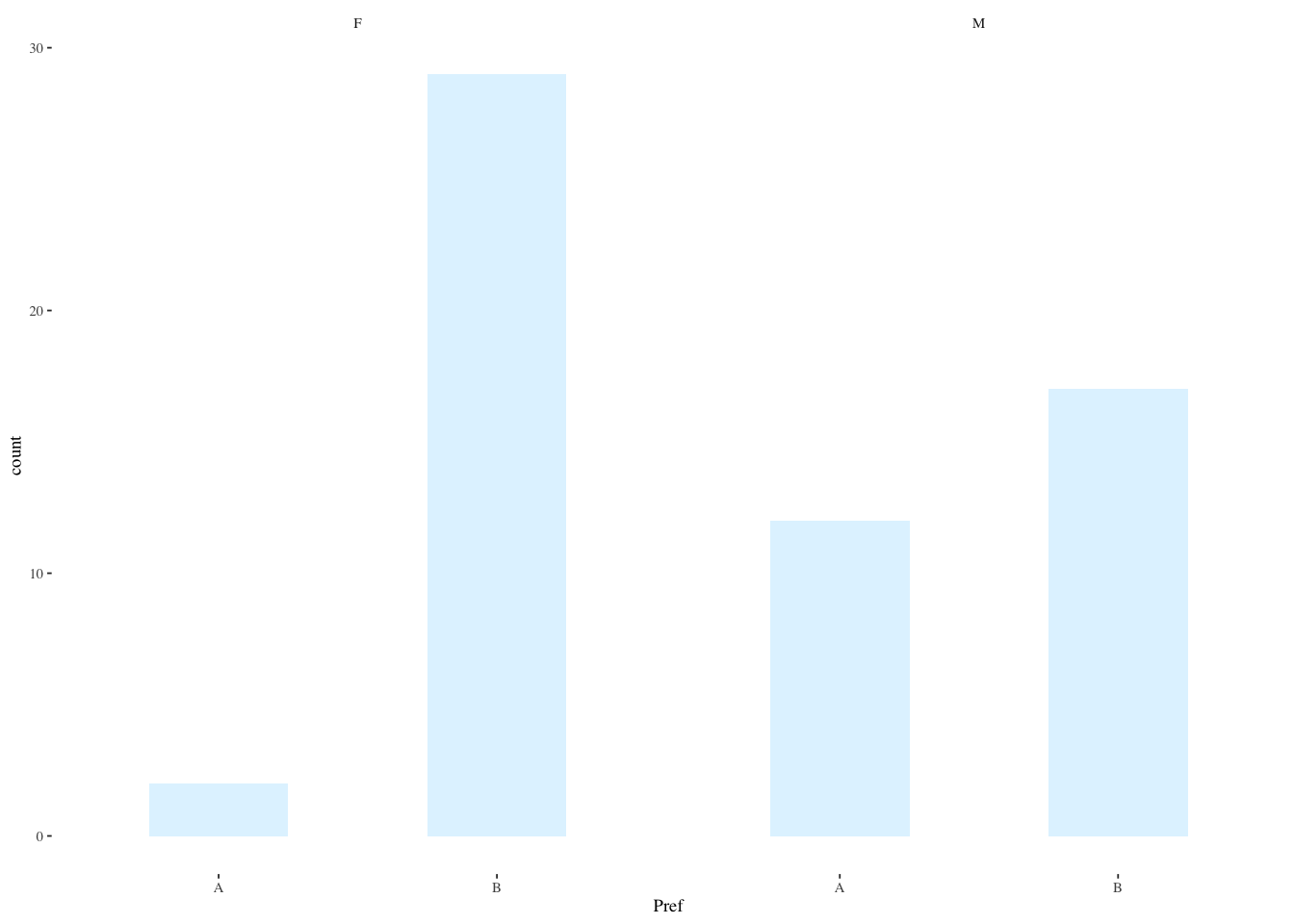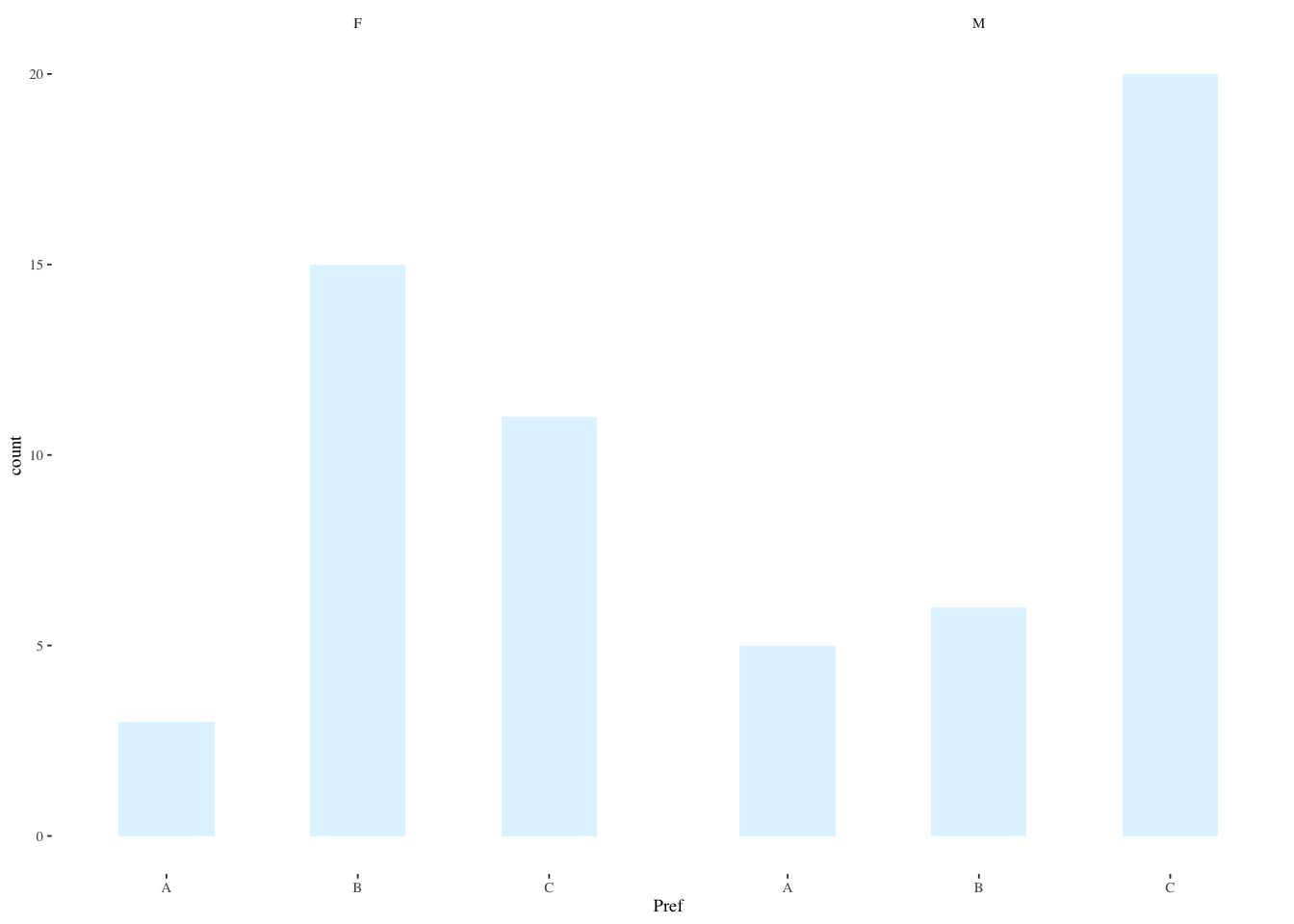# Course NotesonHCI Experiments

Author

Mick McQuaid

Published

April 11, 2023

Following are my course notes from a 2018 Coursera course called Designing, Running, and Analyzing Experiments, taught by Jacob Wobbrock, a prominent HCI scholar. Note that Wobbrock is in no way responsible for any errors or deviations from his presentation.

These course notes are an example of reproducible research and literate programming. They are reproducible research because the same file that generated this html document also ran all the experiments. This is an example of literate programming in the sense that the code, pictures, equations, and narrative are all encapsulated in one file. The source file for this project, along with the data files, are enough for you to reproduce the results and reproduce the documentation. All the source material is available in my github account, although in an obscure location therein.

options(readr.show_col_types=FALSE) # supress column type messages
library(tidyverse)
── Attaching core tidyverse packages ──────────────────────── tidyverse 2.0.0 ──
✔ dplyr     1.1.0     ✔ readr     2.1.4
✔ forcats   1.0.0     ✔ stringr   1.5.0
✔ ggplot2   3.4.1     ✔ tibble    3.1.8
✔ lubridate 1.9.2     ✔ tidyr     1.3.0
✔ purrr     1.0.1
── Conflicts ────────────────────────────────────────── tidyverse_conflicts() ──
ℹ Use the conflicted package (<http://conflicted.r-lib.org/>) to force all conflicts to become errors
library(ggthemes)

# How many prefer this over that? (Tests of proportions)

## How many prefer website A over B? (One sample test of proportions in two categories)

Sixty subjects were asked whether they preferred website A or B. Their answer and a subject ID were recorded. Read the data and describe it.

prefsAB <- read_csv("prefsAB.csv")
tail(prefsAB) # displays the last few rows of the data frame
# A tibble: 6 × 2
Subject Pref
<dbl> <chr>
1      55 A
2      56 B
3      57 A
4      58 B
5      59 B
6      60 A    
prefsAB$Subject <- factor(prefsAB$Subject) # convert to nominal factor
prefsAB$Pref <- factor(prefsAB$Pref) # convert to nominal factor
summary(prefsAB)
    Subject   Pref
1      : 1   A:14
2      : 1   B:46
3      : 1
4      : 1
5      : 1
6      : 1
(Other):54         
ggplot(prefsAB,aes(Pref)) +
geom_bar(width=0.5,alpha=0.4,fill="lightskyblue1") +
theme_tufte(base_size=7)Is the difference between preferences significant? A default $$\chi^2$$ test examines the proportions in two bins, expecting them to be equally apportioned.

To do the $$\chi^2$$ test, first crosstabulate the data with xtabs().

#. Pearson chi-square test
prfs <- xtabs( ~ Pref, data=prefsAB)
prfs # show counts
Pref
A  B
14 46 
chisq.test(prfs)

Chi-squared test for given probabilities

data:  prfs
X-squared = 17.067, df = 1, p-value = 3.609e-05

We don’t really need an exact binomial test yet because the $$\chi^2$$ test told us enough: that the difference is not likely due to chance. That was only because there are only two choices. If there were more than two, we’d need a binomial test for every pair if the $$\chi^2$$ test turned up a significant difference. This binomial test just foreshadows what we’ll need when we face three categories.

#. binomial test
#. binom.test(prfs,split.table=Inf)
binom.test(prfs)

Exact binomial test

data:  prfs
number of successes = 14, number of trials = 60, p-value = 4.224e-05
alternative hypothesis: true probability of success is not equal to 0.5
95 percent confidence interval:
0.1338373 0.3603828
sample estimates:
probability of success
0.2333333 

## How many prefer website A, B, or C? (One sample test of proportions in three categories)

First, read in and describe the data. Convert Subject to a factor because R reads any numerical data as, well, numeric, but we don’t want to treat it as such. R interprets any data with characters as a factor. We want Subject to be treated as a factor.

prefsABC <- read_csv("prefsABC.csv")
head(prefsABC) # displays the first few rows of the data frame
# A tibble: 6 × 2
Subject Pref
<dbl> <chr>
1       1 C
2       2 C
3       3 B
4       4 C
5       5 C
6       6 B    
prefsABC$Subject <- factor(prefsABC$Subject)
prefsABC$Pref <- factor(prefsABC$Pref)
summary(prefsABC)
    Subject   Pref
1      : 1   A: 8
2      : 1   B:21
3      : 1   C:31
4      : 1
5      : 1
6      : 1
(Other):54         
par(pin=c(2.75,1.25),cex=0.5)
ggplot(prefsABC,aes(Pref))+
geom_bar(width=0.5,alpha=0.4,fill="lightskyblue1")+
theme_tufte(base_size=7)You can think of the three websites as representing three bins and the preferences as filling up those bins. Either each bin gets one third of the preferences or there is a discrepancy. The Pearson $$\chi^2$$ test functions as an omnibus test to tell whether there is any discrepancy in the proportions of the three bins.

prfs <- xtabs( ~ Pref, data=prefsABC)
prfs # show counts
Pref
A  B  C
8 21 31 
chisq.test(prfs)

Chi-squared test for given probabilities

data:  prfs
X-squared = 13.3, df = 2, p-value = 0.001294

A multinomial test can test for other than an even distribution across bins. Here’s an example with a one third distribution in each bin.

if (!require(XNomial)) {
install.packages("XNomial",dependencies=TRUE)
library(XNomial)
}
Loading required package: XNomial
xmulti(prfs, c(1/3, 1/3, 1/3), statName="Prob")

P value (Prob) = 0.0008024

Now we don’t know which pair(s) differed so it makes sense to conduct post hoc binomial tests with correction for multiple comparisons. The correction, made by p.adjust(), is because the more hypotheses we check, the higher the probability of a Type I error, a false positive. That is, the more hypotheses we test, the higher the probability that one will appear true by chance. Wikipedia has more detail in its “Multiple Comparisons Problem” article.

Here, we test separately for whether each one has a third of the preferences.

aa <- binom.test(sum(prefsABC$Pref == "A"), nrow(prefsABC), p=1/3) bb <- binom.test(sum(prefsABC$Pref == "B"),
nrow(prefsABC), p=1/3)
cc <- binom.test(sum(prefsABC$Pref == "C"), nrow(prefsABC), p=1/3) p.adjust(c(aa$p.value, bb$p.value, cc$p.value), method="holm")
 0.001659954 0.785201685 0.007446980

The adjusted $$p$$-values tell us that A and C differ significantly from a third of the preferences.

## How many males vs females prefer website A over B? (Two-sample tests of proportions in two categories)

Revisit our data file with 2 response categories, but now with sex (M/F).

prefsABsex <- read_csv("prefsABsex.csv")
tail(prefsABsex)
# A tibble: 6 × 3
Subject Pref  Sex
<dbl> <chr> <chr>
1      55 A     M
2      56 B     F
3      57 A     M
4      58 B     M
5      59 B     M
6      60 A     M    
prefsABsex$Subject <- factor(prefsABsex$Subject)
prefsABsex$Pref <- factor(prefsABsex$Pref)
prefsABsex$Sex <- factor(prefsABsex$Sex)
summary(prefsABsex)
    Subject   Pref   Sex
1      : 1   A:14   F:31
2      : 1   B:46   M:29
3      : 1
4      : 1
5      : 1
6      : 1
(Other):54                

Plotting is slightly more complicated by the fact that we want to represent two groups. There are many ways to do this, including stacked bar charts, side-by-side bars, or the method chosen here, using facet_wrap(~Sex) to cause two separate plots based on Sex to be created.

ggplot(prefsABsex,aes(Pref)) +
geom_bar(width=0.5,alpha=0.4,fill="lightskyblue1") +
facet_wrap(~Sex) +
theme_tufte(base_size=7)Although we can guess by looking at the above plot that the difference for females is significant and the difference for males is not, a Pearson chi-square test provides some statistical evidence for this hunch.

prfs <- xtabs( ~ Pref + Sex, data=prefsABsex) # the '+' sign indicates two vars
prfs
    Sex
Pref  F  M
A  2 12
B 29 17
chisq.test(prfs)

Pearson's Chi-squared test with Yates' continuity correction

data:  prfs
X-squared = 8.3588, df = 1, p-value = 0.003838

## What if the data are lopsided? (G-test, alternative to chi-square)

Wikipedia tells us that the $$G$$-test dominates the $$\chi^2$$ test when $$O_i>2E_i$$ in the formula

$\chi^2=\sum_i \frac{(O_i-E_i)^2}{E_i}$

where $$O_i$$ is the observed and $$E_i$$ is the expected proportion in the $$i$$th bin. This situation may occur in small sample sizes. For large sample sizes, both tests give the same conclusion. In our case, we’re on the borderline for this rule in the bin where 29 females prefer B. All females would have to prefer B for the rule to dictate a switch to the $$G$$-test.

if (!require(RVAideMemoire)) {
install.packages("RVAideMemoire",dependencies=TRUE)
library(RVAideMemoire)
}
Loading required package: RVAideMemoire
*** Package RVAideMemoire v 0.9-81-2 ***
G.test(prfs)

G-test

data:  prfs
G = 11.025, df = 1, p-value = 0.0008989
#. Fisher's exact test
fisher.test(prfs)

Fisher's Exact Test for Count Data

data:  prfs
p-value = 0.001877
alternative hypothesis: true odds ratio is not equal to 1
95 percent confidence interval:
0.009898352 0.537050159
sample estimates:
odds ratio
0.1015763 

## How many males vs females prefer website A, B, or C? (Two-sample tests of proportions in three categories)

Revisit our data file with 3 response categories, but now with sex (M/F).

prefsABCsex <- read_csv("prefsABCsex.csv")
head(prefsABCsex)
# A tibble: 6 × 3
Subject Pref  Sex
<dbl> <chr> <chr>
1       1 C     F
2       2 C     M
3       3 B     M
4       4 C     M
5       5 C     M
6       6 B     F    
prefsABCsex$Subject <- factor(prefsABCsex$Subject)
prefsABCsex$Pref <- factor(prefsABCsex$Pref)
prefsABCsex$Sex <- factor(prefsABCsex$Sex)
summary(prefsABCsex)
    Subject   Pref   Sex
1      : 1   A: 8   F:29
2      : 1   B:21   M:31
3      : 1   C:31
4      : 1
5      : 1
6      : 1
(Other):54                
ggplot(prefsABCsex,aes(Pref)) +
geom_bar(width=0.5,alpha=0.4,fill="lightskyblue1") +
facet_wrap(~Sex) +
theme_tufte(base_size=7)#. Pearson chi-square test
prfs <- xtabs( ~ Pref + Sex, data=prefsABCsex)
prfs
    Sex
Pref  F  M
A  3  5
B 15  6
C 11 20
chisq.test(prfs)
Warning in chisq.test(prfs): Chi-squared approximation may be incorrect

Pearson's Chi-squared test

data:  prfs
X-squared = 6.9111, df = 2, p-value = 0.03157
#. G-test
G.test(prfs)

G-test

data:  prfs
G = 7.0744, df = 2, p-value = 0.02909
#. Fisher's exact test
fisher.test(prfs)

Fisher's Exact Test for Count Data

data:  prfs
p-value = 0.03261
alternative hypothesis: two.sided

Now conduct manual post hoc binomial tests for (m)ales—do any prefs for A–C significantly differ from chance for males?

ma <- binom.test(sum(prefsABCsex[prefsABCsex$Sex == "M",]$Pref == "A"),
nrow(prefsABCsex[prefsABCsex$Sex == "M",]), p=1/3) mb <- binom.test(sum(prefsABCsex[prefsABCsex$Sex == "M",]$Pref == "B"), nrow(prefsABCsex[prefsABCsex$Sex == "M",]), p=1/3)
mc <- binom.test(sum(prefsABCsex[prefsABCsex$Sex == "M",]$Pref == "C"),
nrow(prefsABCsex[prefsABCsex$Sex == "M",]), p=1/3) #. correct for multiple comparisons p.adjust(c(ma$p.value, mb$p.value, mc$p.value), method="holm")
 0.109473564 0.126622172 0.001296754

Next, conduct manual post hoc binomial tests for (f)emales—do any prefs for A–C significantly differ from chance for females?

fa <- binom.test(sum(prefsABCsex[prefsABCsex$Sex == "F",]$Pref == "A"),
nrow(prefsABCsex[prefsABCsex$Sex == "F",]), p=1/3) fb <- binom.test(sum(prefsABCsex[prefsABCsex$Sex == "F",]$Pref == "B"), nrow(prefsABCsex[prefsABCsex$Sex == "F",]), p=1/3)
fc <- binom.test(sum(prefsABCsex[prefsABCsex$Sex == "F",]$Pref == "C"),
nrow(prefsABCsex[prefsABCsex$Sex == "F",]), p=1/3) #. correct for multiple comparisons p.adjust(c(fa$p.value, fb$p.value, fc$p.value), method="holm")
 0.02703274 0.09447821 0.69396951

# How do groups compare in reading performance? (Independent samples $$t$$-test)

Here we are asking which group read more pages on a particular website.

pgviews <- read_csv("pgviews.csv")
pgviews$Subject <- factor(pgviews$Subject)
pgviews$Site <- factor(pgviews$Site)
summary(pgviews)
    Subject    Site        Pages
1      :  1   A:245   Min.   : 1.000
2      :  1   B:255   1st Qu.: 3.000
3      :  1           Median : 4.000
4      :  1           Mean   : 3.958
5      :  1           3rd Qu.: 5.000
6      :  1           Max.   :11.000
(Other):494                           
tail(pgviews)
# A tibble: 6 × 3
Subject Site  Pages
<fct>   <fct> <dbl>
1 495     A         3
2 496     B         6
3 497     B         6
4 498     A         3
5 499     A         4
6 500     B         6
#. descriptive statistics by Site
plyr::ddply(pgviews, ~ Site, function(data) summary(data\$Pages))
  Site Min. 1st Qu. Median     Mean 3rd Qu. Max.
1    A    1       3      3 3.404082       4    6
2    B    1       3      4 4.490196       6   11
plyr::ddply(pgviews, ~ Site, summarise, Pages.mean=mean(Pages), Pages.sd=sd(Pages))
  Site Pages.mean Pages.sd
1    A   3.404082 1.038197
2    B   4.490196 2.127552
#. graph histograms and a boxplot
ggplot(pgviews,aes(Pages,fill=Site,color=Site)) +
geom_bar(alpha=0.5,position="identity",color="white") +
scale_color_grey() +
scale_fill_grey() +
theme_tufte(base_size=7)